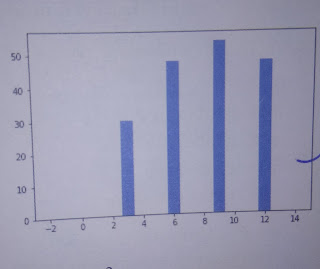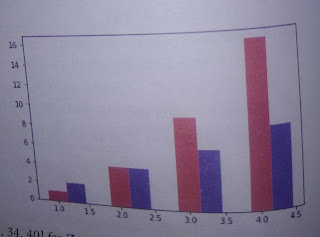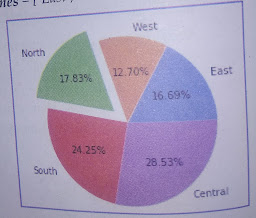Contents

## data visualization using pyplot important questions

### Q 1. name some commonly used chart types.

ans. some commonly used charts types are line chart,bar chart,pie chart,scatter chart etc.

### Q 2. name the function you will use to create a (i)line chart,(ii)bar chart,(iii)pie chart?

ans. (i) matplotlib.pyplot.plot()
(ii) matplotlib.pyplot.bar()
(iii) matplotlib.pyplot.pie()

### Q 3. what is python’s support data visualization?

ans. python’s support data visualization by providing some useful libraries for visualization.most commonly used data visualization library is matplotlib .
matplotlib is a python library,also sometimes known as plotting library.
there are many other libraries of python that can be used for data visualization but matplotlib is very popular for 2D plottting.

### Q 4. what is pylot?is a python library?

ans. the pyplot is one of the interfaces of matplotlib library of pyton.this interface offers simple  MATLAB style functions that can be used for plotting various types of charts using underlying matplotlib library’s functionality.
pyplot are interface,collection of methods for accessing and using underlying functionality a library,not a library.the matplotlib library has may other interfaces too,along with pyplot interface.

### Q 5. consider the code below (all required libraries are imported) and the output produced by it.why is the chart showing one bar only while we are plotting four values on the chart.

a=[3,6,9,12]
b=[30,48,54,48]
plt.xlim(-3,5)
plt.bar(a,b)
plt.show()
ans. the given chart is showing a single bar as the limits of x axis have been set -3 to 5.on this range, only one value from the data range being plotted falls,only a and b fall on this range.thus only a single value b i.e,30 is plotted against a i.e,3.

### Q 6. what changes will you make to the code of previous question so that the bars are visible for all four points.but do keep in mind that the x axis must begin from the point -3.

ans.  a=[3,6,9,12]
b=[30,48,54,48]
plt.xlim(-3,15)
plt.bar(a,b)
plt.show()data visualization using pyplot important question

### Q 7. why is following code not producing any result? why is it giving error. (note: all required library have been imported and are    available)

a= range(10,50,12)
b= range(90,200,20)
matplotlib.pyplot.plot(a,b)
ans. the above code is producing error because the two sequences being plotted i.e,a and b do not match in shape.while sequence ‘a’ contains 4 elements,sequence ‘b’ contains 6 elements.for plotting,it is necessary that the two sequences being plotted must match in their shape.

### Q 8. given an ndarray p as ([1,2,3,4]).write code to plot a bar chart having bars for p and p**2 (with red color) and another bar for p*2 (with blue color).(assume that libraries have been imported)

ans. plt.bar(p,p**2,color= ‘r’,width=0.3)
plt.bar(p+0.3,p*2,color= ‘b’,width=0.3)double bar chart

### Q9. write to create a pie for the sequences con=[23.4,17.8,25,34,40] for zones=[‘east’,’west’,’north’,’south’]. *show north zone’s value exploded *show % contribution for each zone *the pie chart should be circular.

ans.
import matplot.pyplot as plt
con=[23.4,17.8,25,34,40]
zones=[‘east’,’west’,’north’,’south’,’central’]
plt.axis(“equal”)
plt.pie(con,labels=zones,explode=[0,0,0.2,0,0],autopct=”%  1.2f%%”)double bar chart

### Q 10. what do you understand by numpy.

ans.
numpy:- is the core library for scientific computing in python.it provides a high performance,multidimensional array object and tools for working with these arrays.
module to be imported:- import numpy as np .

### Q 11. what setting would you add to print a circular pie chart?assume that pyplot library has been imported as gr.

ans. gr.axis(‘equal’)

### Q12. why is following command producing error?plt is the alias name of imported library matplot.pyplot and A is an ndarray created as:- A=np.arrange(2,20,2) plt.pie(A,A+2,A+3,A+4)

ans. the pie() function can handle only one data series.